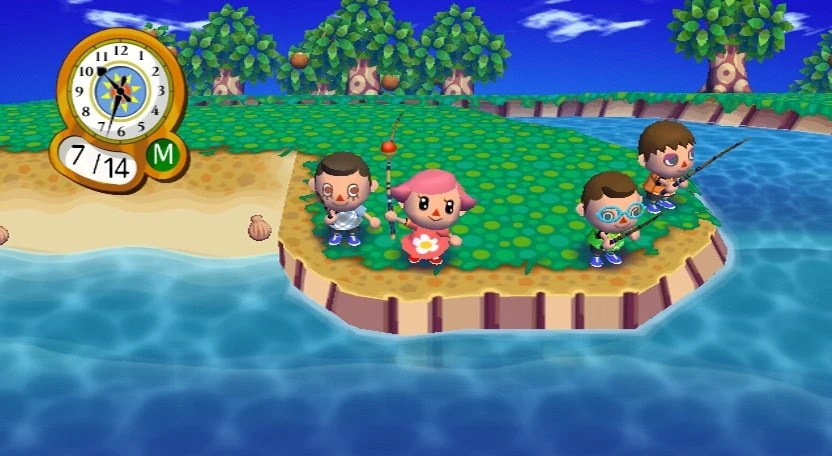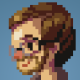## Animal Crossing Curved World Shader

Continuing the theme of my last post, I thought I’d write another shader – this time to mimic the cylindrical rolloff effect of distant objects seen in, amongst others, the “Animal Crossing” series. Rather than appearing on a flat plane, the world surface gently rolls towards you as if projected onto a cylindrical surface, as shown below:The following code reproduces this effect – the comments are fairly explicit as to how it works. Note that rather than being a generic HLSL/Cg pixel-fragment shader, it’s a surface shader, which means it’ll only work in Unity. The advantage, however, is that it’ll automatically calculate lighting and shadow calculations without me having to write any nasty dot products of surface normals compared to light direction etc….

```Shader "Custom/CurvedWorld" {
Properties {
// Diffuse texture
_MainTex ("Base (RGB)", 2D) = "white" {}
// Degree of curvature
_Curvature ("Curvature", Float) = 0.001
}
Tags { "RenderType"="Opaque" }
LOD 200

CGPROGRAM
// Surface shader function is called surf, and vertex preprocessor function is called vert

uniform sampler2D _MainTex;
uniform float _Curvature;

// Basic input structure to the shader function
// requires only a single set of UV texture mapping coordinates
struct Input {
float2 uv_MainTex;
};

// This is where the curvature is applied
void vert( inout appdata_full v)
{
// Transform the vertex coordinates from model space into world space
float4 vv = mul( _Object2World, v.vertex );

// Now adjust the coordinates to be relative to the camera position
vv.xyz -= _WorldSpaceCameraPos.xyz;

// Reduce the y coordinate (i.e. lower the "height") of each vertex based
// on the square of the distance from the camera in the z axis, multiplied
// by the chosen curvature factor
vv = float4( 0.0f, (vv.z * vv.z) * - _Curvature, 0.0f, 0.0f );

// Now apply the offset back to the vertices in model space
v.vertex += mul(_World2Object, vv);
}

// This is just a default surface shader
void surf (Input IN, inout SurfaceOutput o) {
half4 c = tex2D (_MainTex, IN.uv_MainTex);
o.Albedo = c.rgb;
o.Alpha = c.a;
}
ENDCG
}
// FallBack "Diffuse"
}
```

The preceding code gives an effect as follows (these objects are all placed on a flat, level plane – the curvature is applied solely in the shader):The effect is perhaps more satisfying when animated:

Note that, if you want the world to have a spherical rolloff – i.e. to rolloff in both the x and z axes, then adjust the line in the vertex modifier function to be as follows:

```vv = float4( 0.0f, ((vv.z * vv.z) + (vv.x * vv.x)) * - _Curvature, 0.0f, 0.0f )
```

And, if you provide a negative curvature value, the world will apparently curve up towards you instead:

This entry was posted in Game Dev and tagged , , , . Bookmark the permalink.

### 17 Responses to Animal Crossing Curved World Shader

1.Francis Humphries says:

Hey there- I’ve just been playing with this, and I’ve noticed sometimes it culls objects before they’ve actually disappeared. Is there a solution to this?

2.Santosh says:

Hey guys i’m also looking for same result as shown in above screenshot, but i’m not able to attach the shader to camera.

•alastaira says:

You don’t attach the shader to the camera – you apply it to object(s) in the world.

3.Tales Sampaio says:

Oh my god! Works like a charm!
Thank you very much!! =D

4.George says:

Hi
it worked on Editor, but now working on Android device

5.Simon says:

Unfortunately the depth pass is still unchanged, so Post Processing Effects or Projectors dont work as expected.. Any way to have Unity calculate the correct depth pass with the curvature applied?

6.Anitam says:

Hi,
I am working on a project where i need bending with lightmaps and brightness.
How can it be modified to support those ?
Thanks,
Anitam

7.Kiran says:

run great on editor ,but on Android ,im geting pink ground ,also object which have shader .

8.slothprod says:

Thanks for the shader. How can I modify it to bend the world on the X axis and not on the y?

9.Henrique says:

Hi. How did you manage to apply the material to all objects in th scene?

10.Sid says:

Properties {
// Diffuse texture
_MainTex (“Base (RGB)”, 2D) = “white” {}
// Degree of curvature
_Curvature (“Curvature”, Float) = 0.001
// Axis Around which the curvature is required
_Axis (“Axis”, int ) = 2

}
Tags { “RenderType”=”Opaque” }
LOD 200

CGPROGRAM
// Surface shader function is called surf, and vertex preprocessor function is called vert

uniform sampler2D _MainTex;
uniform float _Curvature;
uniform int _Axis;

// Basic input structure to the shader function
// requires only a single set of UV texture mapping coordinates
struct Input {
float2 uv_MainTex;
};

// This is where the curvature is applied
void vert( inout appdata_full v)
{
// Transform the vertex coordinates from model space into world space
float4 vv = mul( _Object2World, v.vertex );

// Now adjust the coordinates to be relative to the camera position
vv.xyz -= _WorldSpaceCameraPos.xyz;

// Reduce the y coordinate (i.e. lower the “height”) of each vertex based
// on the square of the distance from the camera in the z axis, multiplied
// by the chosen curvature factor
if(_Axis == 0)
vv = float4( 0.0f, (vv.x * vv.x) * – _Curvature, 0.0f, 0.0f );
else if(_Axis == 1)
vv = float4( 0.0f, (vv.y * vv.y) * – _Curvature, 0.0f, 0.0f );
else if(_Axis == 2)
vv = float4( 0.0f, (vv.z * vv.z) * – _Curvature, 0.0f, 0.0f );

// Now apply the offset back to the vertices in model space
v.vertex += mul(_World2Object, vv);
}

// This is just a default surface shader
void surf (Input IN, inout SurfaceOutput o) {
half4 c = tex2D (_MainTex, IN.uv_MainTex);
o.Albedo = c.rgb;
o.Alpha = c.a;
}
ENDCG
}
// FallBack “Diffuse”
}

•Sid says:

Added axis for curvature. Now you can bend the world in the direction you want. Cheers 🙂

11.jtpup0 says:

The line “vv = float4( 0.0f, ((vv.z * vv.z) + (vv.x * vv.x)) * – _Curvature, 0.0f, 0.0f )” works as “vv = float4( 0.0f, (vv.z * vv.zv + v.x * vv.x) * – _Curvature, 0.0f, 0.0f )” too

12.Justin says:

Hey, this is quite a nice shader, learned alot from your contribution! I wonder how would you do a “Horizontal Curving” where instead of curving down on the y-axis it curves left/right instead? Thanks!

13.canu1832 says:

14.xuelong says:
15.Pete Loco says: